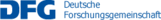## C05 – Discrete Riemannian calculus on shape space

The theory of shape spaces in vision is linked both to concepts from geometry and from physics. The flow of diffeomorphism approach and the optimal transportation approach are prominent examples for Riemannian metric structures on the space of shapes, intensively studied in the last decade. In general, the numerical realization of the underlying infinite dimensional Riemannian calculus poses enormous computational challenges. In this project we propose suitable time and space discrete approximations. They will be based on optimal matching deformations, which are significantly cheaper to compute but by construction non-Riemannian. We will also study abstract concepts of transportation between metric measure spaces with particular focus on spaces consisting only of a fixed number of points.

In the time discrete calculus a discrete path energy is defined as the sum of pairwise deformation energies along a discrete path. Using a variational approach one can deduce from this step by step a discrete logarithm, a discrete exponential map, a discrete parallel transport, a discrete Levi-Civita connection, and finally a discrete Riemannian curvature tensor. This concept will be applied and analyzed in particular for the flow of diffeomorphism and the optimal transportation approach.

Furthermore, with respect to a spatially discrete covering of shape space, the approach of deformation based shape dissimilarities will be combined with the diffusion map paradigm expedited by Coifman and coworkers to introduce physically sound and computationally efficient approximations of metric structures on shape spaces.

Overall, we aim at combining methods from geometry, stochastic analysis and numerics to advance theory in computer vision and explore new applications with efficient computational tools.

Name Institute Location Phone
Rumpf, Martin INS En60/2.035 7866 martin.rumpf@ins.uni-bonn.de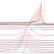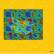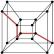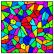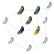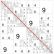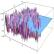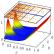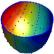## Contact

Coordinator: Prof. Dr. Stefan Müller